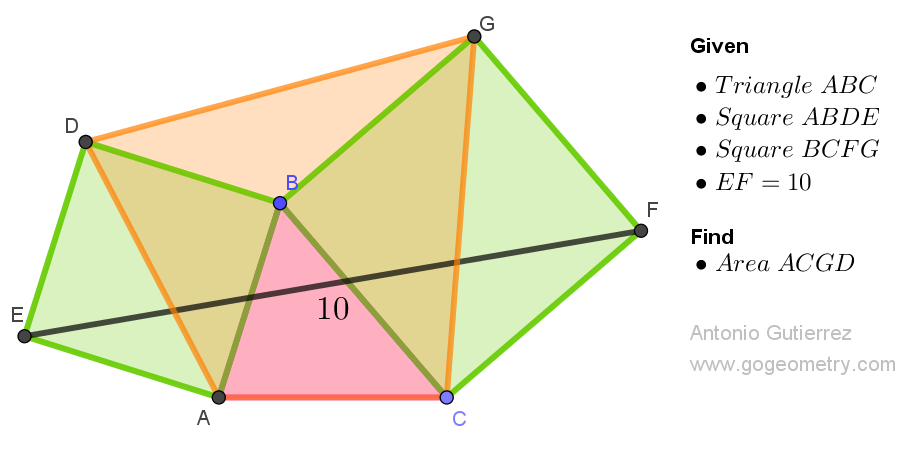# Geometry Problem 1538: Unlocking the Secrets of Triangular Geometry: Solve for the Area of a Quadrilateral Using External Squares and a Segment Length

In triangle ABC, the external squares ABDE and BCFG are constructed on sides AB and BC, respectively. If the length of segment EF is 10 units, what is the area of quadrilateral ACGD?Problem 1538
External squares reveal all,